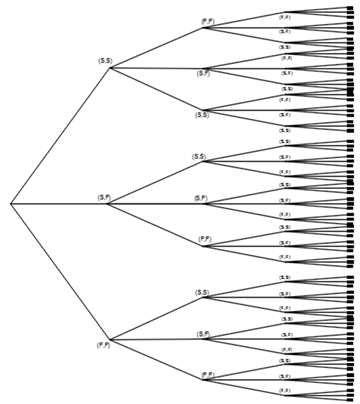Turkish Journal of Analysis and Number Theory
ISSN (Print): 2333-1100 ISSN (Online): 2333-1232
Journal Browser
Article Metrics
• 95
Views
• 292
Saves
• 0
Citations
• 0
Likes
Export Article
Turkish Journal of Analysis and Number Theory. 2020, 8(5), 80-90
DOI: 10.12691/tjant-8-5-1
Open AccessArticle

### Introduction of p-nomial Distribution as a Generalization of Binomial Distribution

1Mathematics and Structural Analysis, Atta Engineering Design Office, El Jadida, Morocco

Pub. Date: September 24, 2020

Cite this paper:
Aziz ATTA. Introduction of p-nomial Distribution as a Generalization of Binomial Distribution. Turkish Journal of Analysis and Number Theory. 2020; 8(5):80-90. doi: 10.12691/tjant-8-5-1

### Abstract

The theory of probability and statistics, thanks to its continuous modernization, has become more and more important in our life given its presence in several fields such as economics and prevision . The binomial distribution is among the oldest probability distributions introduced by Bernoulli . In the same context, we thought of generalizing this probability distribution under the name p-nomial distribution using p-nomial coefficients p-nomial theorem . In this article, we are going to be interested in the introduction of this new probability distribution as well as an establishment of its various standard characteristics. the purpose of this article is therefore summarized in the tracing of the theoretical framework with some examples of application of the said p-nomial distribution.This work is licensed under a Creative Commons Attribution 4.0 International License. To view a copy of this license, visit http://creativecommons.org/licenses/by/4.0/

### FiguresFigure of 7

### References:

  Yadolah Dodge, Statistique, disctionnaire encyclop¨¦dique, Paris 2007.  Anders Hald, A History of Probability and Statistics and Their Applications before 1750, John Wiley & Sons, 2005.  Eric Gossett, Discrete Mathematics with Proof, John Wiley & Sons, 2009.  Michiel Hazewinkel, Encyclopaedia of Mathematics, vol. 2, Springer Science+Business Media, 1994.  Norman Johnson, Adrienne Kemp and Samuel Kotz, Univariate Discrete Distributions, John Wiley & Sons, 2005  Alan Ruegg, Probabilit¨¦s et statistique, vol. 3, PPUR, 1994.  Aziz ATTA, "p-nomial Coefficients and p-nomial Theorem." Turkish Journal of Analysis and Number Theory, vol. 8, no. 1 (2020): 6-15.  F.M. Dekking C. Kraaikamp H.P. Lopuhaa L.E. Meester, A Modern Introduction to Probability and Statistics, Understanding Why and How, Delft Institute of Applied Mathematics Delft University of Technology Mekelweg 4 2628 CD Delft The Netherlands.  Charles M. Grinstead, J. Laurie Snell, Introduction to Probability, Swarthmore College and Dartmouth College, USA.  Aziz ATTA, "Twin Polynomials and Kernels Matrix." Turkish Journal of Analysis and Number Theory, vol. 8, no. 3 (2020): 57-69.  Andreas N. Philippou, Demetrios L. Antzoulakos, Binomial Distribution, Chapter ¡¤ January 2011,  Matthew Aldridge, Measure Theory and Integration: Measures, MA40042, UK.  M. Samuel Fiorini, MATH-F-307 Math¨¦matiques discr¨¨tes, Version du 5 octobre 2012.